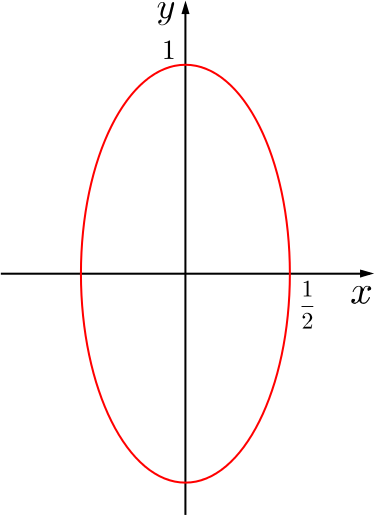Review question

# Given this log equation, when can $a$ be largest? Add to your resource collection Remove from your resource collection Add notes to this resource View your notes for this resource

Ref: R7015

## Solution

Given that $a$ and $b$ are positive and $4(\log_{10}a)^2+(\log_{10}b)^2=1$ then the greatest possible value of $a$ is

1. $\frac{1}{10}$,

2. $1$,

3. $\sqrt{10}$,

4. $10^{\sqrt{2}}$.

#### Approach 1

Note first that $a$ is largest when $\log_{10}a$ is largest, since $\log$ is an increasing function. So $a$ is largest when $(\log_{10}b)^2$ is smallest.

For positive $b$, the minimum value that $(\log_{10}b)^2$ can take is $0$ (when $b=1$). Then we have $4(\log_{10}a)^2=1,$ which is equivalent to $\log_{10}a =\pm \frac{1}{2}.$

So the largest value this takes is $\log_{10}a = \frac{1}{2}$, which is when $a=\sqrt{10}$.

In fact, this also shows that the minimum value for $\log_{10}a$ happens when $b = 1$ and $\log_{10}a = -1/2$. That is, when $a = 1/\sqrt{10}$.The ellipse $4x^2 + y^2 = 1$

#### Approach 2

Alternatively, we could take a geometrical approach. Let $x = \log_{10}a$, $y = \log_{10}b$. The maximum value for $x=\log_{10}a$ is achieved when $a$ takes its maximum value, since $\log$ is an increasing function.

Our equation now becomes $4x^2 + y^2 = 1$, which is an ellipse.

From the sketch, we can see that $x$ has maximum $\frac{1}{2}$ and so $a$ has maximum $\sqrt{10}$, by the same calculation as above.

As before, the answer must be (c).